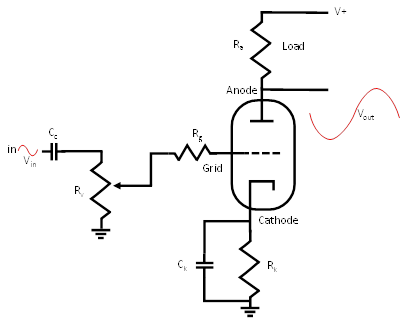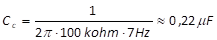The input stage of an integrated vacuum tube amplifier is basically a voltage amplifier, which was already discussed in Chapter 3:.

An example of input stage is shown in Figure 27. A resistive load Ra is connected to the anode of the vacuum tube. The output voltage is taken at the anode itself, before the load. The grid bias voltage for the input stage is obtained by using self-biasing with a cathode resistor Rk at the vacuum tube cathode. The cathode resistor is bypassed by a capacitor Ck to reduce the local negative feedback, produced by the cathode resistor, and to increase gain. Cathode biasing and local negative feedback details were already discussed in Section 3.6.2.Figure 27: Basic schema of the input stage of an integrated vacuum tube amplifier.The input stage is basically a voltage amplifier. It takes the input signal, coming from an external source, and applies it to the grid of the vacuum tube. Cathode bias is generally used in this stage, and the cathode resistor is generally bypassed by a capacitor to reduce the local negative feedback and increase the gain.

Next example shows how to draw the loadline, set the quiescent operating point, and chose the cathode resistor for self-biasing the input stage.

 Example 12: Loadline and bias of the input stage Suppose, for instance, the input stage is built using a 12AX7 vacuum tube, the high-tension V+ is 300 V, and the load Ra is 220K Ohm. When the vacuum does not conduce, the anode to cathode voltage is 300V. In the theoretical case that the vacuum tube does not offer any resistance, the anode current is 300V/220K Ohm=1.35mA. By connecting these two points, we obtain the loadline plotted in Figure 28. A good operating point is identified by the red spot. It corresponds to a bias current Ib of 0.65mA and an anode to cathode voltage of Va 160V. This can be obtained with a grid bias voltage of -1.5V. Since we are using cathode biasing, the grid is at ground level and we have to elevate the cathode voltage to 1.5V, by computing an appropriate value of the cathode resistor Rk. By using the Ohm law, we have that Rk=Vk/Ib=1.5V/0.65mA=2.3K Ohm. The closest standard resistance is 2.2K Ohm, which is a good approximation.

The bypass capacitor Ck has the purpose, as discussed in Section 3.6.2, to reduce local negative feedback and increase gain. Small capacitance values increase gain just for high frequencies, high ones increase gain also for low frequencies. For instance, in our case, using the calculations discussed in Section 3.6.3, we determine that a value of 100μF is enough to bypass and increase gain at all audible frequencies.

The grid stopper resistor Rg is used to block very high frequencies that can enter the circuit and parasitic oscillations, by forming a low-pass filter with the internal vacuum tube capacitance. As we already said, values around 47K Ohm are generally used with 12AX7 vacuum tubes.

The use of a grid stopper resistor is particularly important at the input stage of an integrated vacuum tube amplifier. We are at the very beginning of the amplifier stages and signals that have to be amplified are very small. All noises, interferences, parasitic oscillations are here significantly amplified through all the remaining stages. For instance, consider that the wire connecting the input jack to the gird cannot be generally very short, for practical assembling issues. Therefore, it acts as an antenna, capturing electromagnetic interferences, which must be blocked before being amplified.

The potentiometer Rv is used to control the volume of the amplifier, that is the amount of input signal applied to the grid of the input stage vacuum tube. The potentiometer also acts as a grid leak resistor and forms, with the coupling capacitor Cc, a high-pass filter that blocks the unwanted low frequencies. The coupling capacitor Cc also isolates the input stage from possible DC coming from the external input source.

 Example 13: Coupling capacitor of the input stage of an integrated vacuum tube amplifier The value of the coupling capacitor Cc can be computed using the high pass filter formula. Suppose the Rv potentiometer is 100k ohm, and we want to stop all frequencies below 7 Hz. We have:.